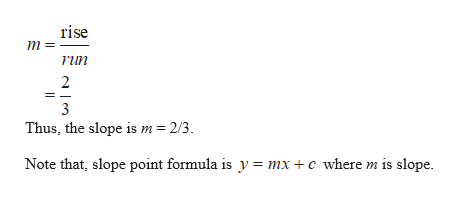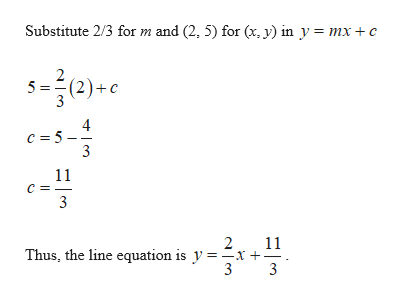# Determine two or more ordered pairs for each relation. THe rise is 2, the run is 3; (2,5) lies on the line.

Question
18 views

Determine two or more ordered pairs for each relation. THe rise is 2, the run is 3; (2,5) lies on the line.

check_circle

Step 1

It is given that, the rise is 2, the run is 3 and point (2, 5) lies on the line.

Obtain the slope as follows:help_outlineImage Transcriptioncloserise m run 2 3 Thus, the slope is m = 2/3 Note that, slope point formula is y = mx +c where m is slope fullscreen
Step 2

Obtain the value of c a...help_outlineImage TranscriptioncloseSubstitute 2/3 for m and (2, 5) for (x, y) in y = mx + c 512)c c 5 11 c 11 Thus, the line equation is y = -x+ 3 2 fullscreen

### Want to see the full answer?

See Solution

#### Want to see this answer and more?

Solutions are written by subject experts who are available 24/7. Questions are typically answered within 1 hour.*

See Solution
*Response times may vary by subject and question.
Tagged in